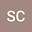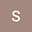Eyring-Powell non-Newtonian fluid flow with Joule heating and thermal radiation effects: A numerical study.
••• Sulochana C,
• * Savita
Sulochana C
Gulbarga University

Corresponding Author:[email protected]

Author Profile* Savita
Gulbarga University
Author Profile## Abstract

The Eyring-Powell non-Newtonian fluid model is widely used to described flow behavior with lowest and highest shear rates. Statistical analysis is carried out in this present article to explore non-Newtonian BL flow past an elongating sheet which is moving with linear velocity. Similarity transforms are applied to express fluid governing equations as ODEs which are then solved by bvp4c algorithm in MATLAB software. In the energy equation thermal radiation and joule heating effects are considered. Important key factors such as Nusselt number and friction at the surface are computed with respect to different influencing fluid parameters.